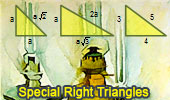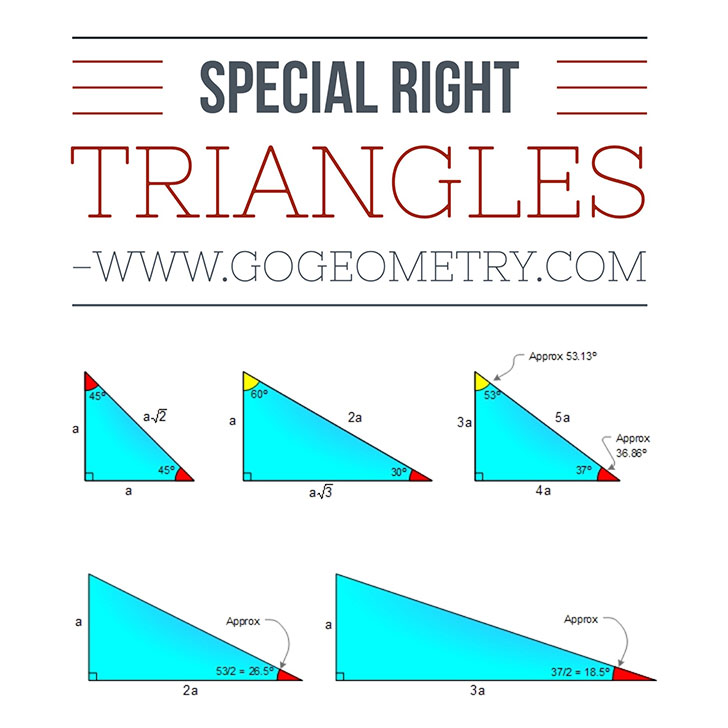# Mastering Geometry: Exploring Special Right Triangles, Theorems, and Problem SolvingA special right triangle  is a right triangle with some regular feature that makes calculations on the triangle easier, or for which simple formulas exist. Angle based right triangle: 30-60-90, 45-45-90. (Angles that form a simple ratio) Side based right triangle: 3-4-5 (The lengths of the sides form a whole number ratio), approx angles 37-53. Knowing the ratios of the angles or sides of these special right triangles allows one to quickly calculate various lengths in geometric problems without resorting to more advanced methods.Special right triangles have many benefits when it comes to solving geometry problems. Some of these benefits include: Simplifying calculations: Special right triangles have specific ratios of their sides, making them easier to work with and simplifying calculations. For example, in a 45-45-90 triangle, the ratio of the length of the legs to the length of the hypotenuse is 1:1:√2, which can make calculations involving the sides of the triangle much simpler. Facilitating problem-solving: Recognizing a special right triangle in a geometry problem can make it much easier to solve. By understanding the properties of special right triangles and how they relate to other geometric concepts, students can quickly identify the relevant information in a problem and use it to find the solution. Building a foundation for advanced math: Special right triangles are a fundamental concept in geometry and lay the groundwork for more advanced mathematical concepts, such as trigonometry and calculus. By mastering special right triangles, students can build a strong foundation for these more complex subjects. Providing a real-world context: Special right triangles are used in many real-world applications, such as in architecture and engineering. By learning about special right triangles, students can gain a better understanding of how math is used in the real world and how it relates to their everyday lives. Overall, special right triangles are a powerful tool in solving geometry problems. By understanding their properties and how they relate to other geometric concepts, students can simplify calculations, solve problems more efficiently, and build a strong foundation for advanced math.